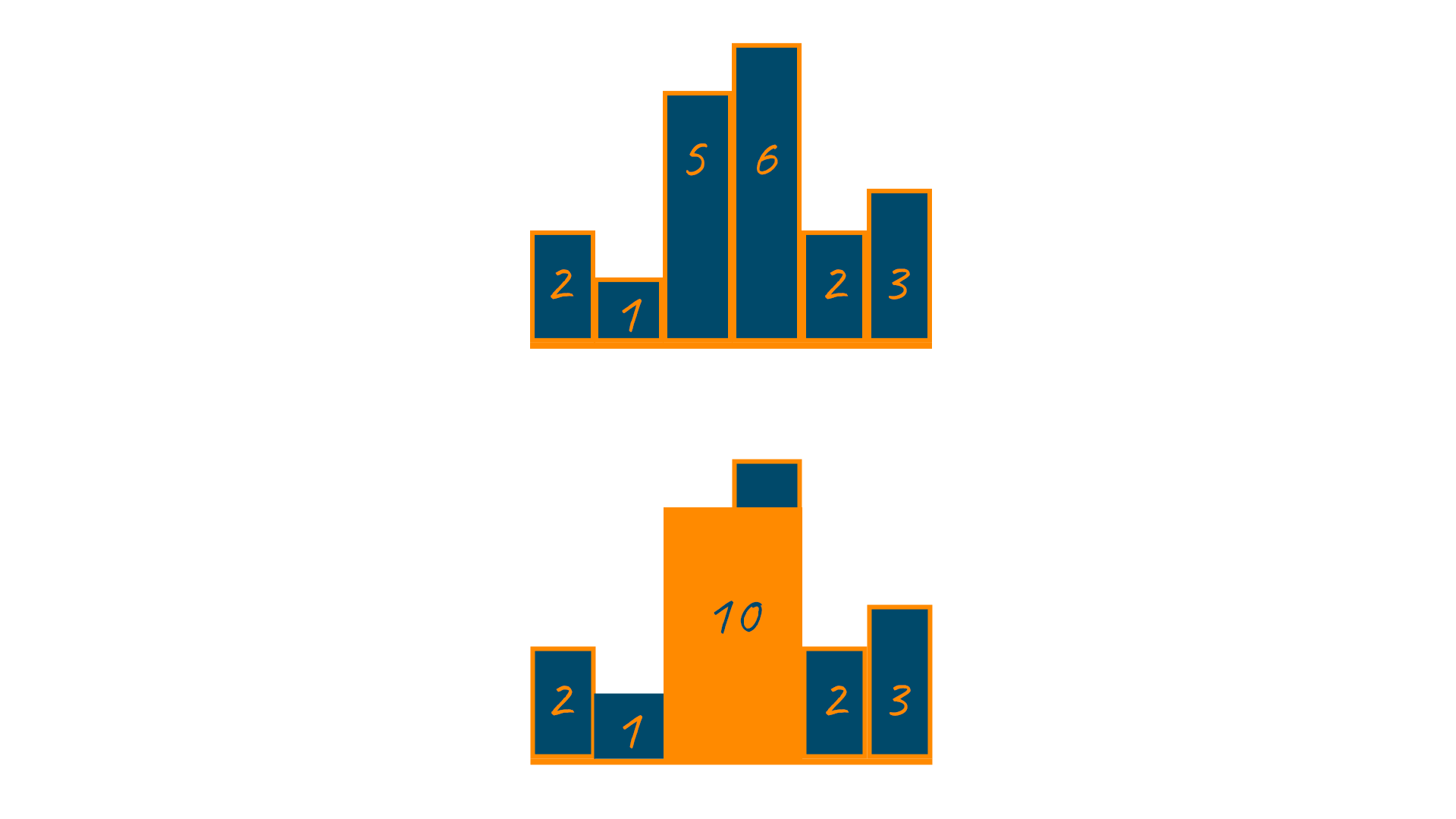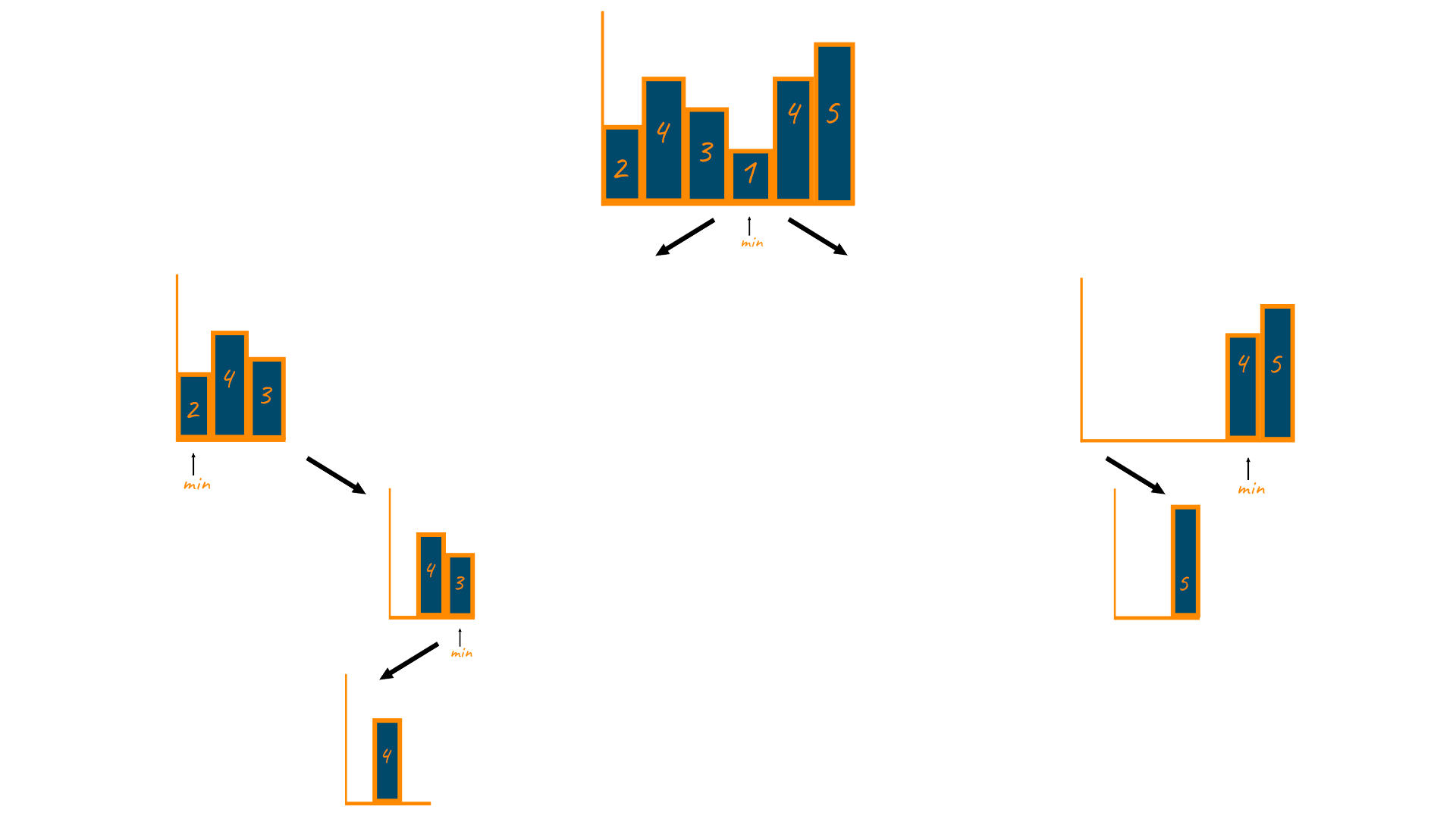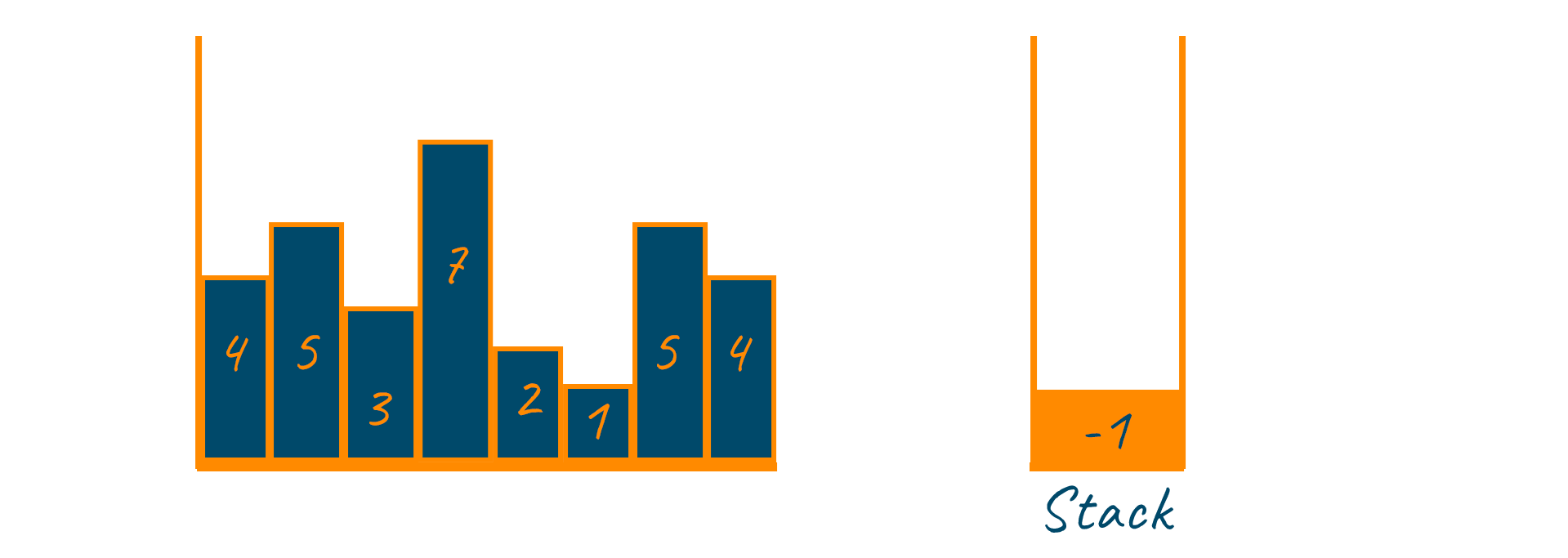# Largest Rectangle in Histogram

It is widely agreed that technical interviews for product-based companies, such as Microsoft and Amazon, can be difficult to pass. You require lots of practice with competitive problems and technical knowledge to get into your dream company. To help you with the same, here we present one such competitive problem that is “Largest Rectangle in Histogram” mostly asked during interviews for Facebook, Amazon, and Google. Here we understand the problem statement and basic approaches to solving this problem using their algorithms and implementation. So, let's begin!

## Problem Statement

Given an array of integer heights representing the histogram’s bar height where the width of each bar is 1, return the area of the largest rectangle in the histogram.

Note that a histogram is a graphical display of numerical data using rectangles of different heights. The rectangles are arranged in the same order as the corresponding elements in the array.

### For example:

Heights = [2, 1, 5, 6, 2, 3]Output: 10

## Solutions

Below are three approaches by which you can find the largest rectangle in the histogram. Let us understand in detail below:

### Method 1: Using the brute force approach

The key idea to take away is that the height of the maximum area between any two bars will always be bounded by the height of the shortest bar lying between them. Below is an image demonstrating this concept.#### Algorithm:

```We first create a variable, ‘maximumArea’, and assign it the value of the maximum area of the histogram.
Later, run two nested loops: one counting from 0 until i = N, and the other counting from j = 0 to j = N.
For each ‘jth’ iteration of this loop, we count ‘k’ from i = k until k = j and find the height of the lowest bar among them.
The area of the histogram would be ‘mininumHeight * (j-i+1)’
Maximize ‘maximumArea’
```

#### Time Complexity

The algorithm's time complexity is O(N^3), where N is the size of the array. Similarly, the space complexity of the above problem is O(1) as no extra space is required while using this approach.

### Method 2: Using the divide & conquer approach

The divide-and-conquer algorithm is designed to avoid traversing through every element in two arrays. Therefore, instead of traversing through every element from A[i] and A[j], you can apply the divide and conquer algorithm as shown in the below image.If you clearly observe the above image, you’ll identify the below two outcomes:

• The rectangle with the greatest area has a width and a height equal to the shortest bar.
• The area of the rectangle formed on the right and left side of the minimum height

As you have divided your problem, you need to conquer the solution simply depending on the recursion.

#### Time Complexity

The time complexity for the divide and conquer approach for finding the largest rectangle in the histogram is O(NlogN), where N is the size of the array. similarly, the space complexity of the approach is O(N) as the approach uses the extra array to find the area.

### Method 3: Using the stack data structure

The concept is similar to stack-based searching for the Next Greater element. Instead of looking for the next larger element, we will use two arrays left[] and right[] to represent the smaller elements on the left and right respectively. For a better understanding, have a look at the below image:#### Algorithm:

```Initialize stack ‘S’ and push the top index of A[] onto ‘S’
Traverse via A[] and compare the height of each element with the height of each element on top of ‘S’
If A[i]>= A[S.top()], then push it into the S
Else A[i] <= A[S.top()], then pop the elements until A[i]>= A[S.top()]
This ensures that A[S.top()] stays at its maximum height as elements are being added to it from above and below
Lastly, push i for every item currently on S into the stack, followed by popping from S until S has no elements left in it.
Return maximum element
```

#### C++ Code for Finding Largest Rectangle in Histogram

```#include
#include
#include
#include
#include  //For using min/max function.
//Above are the libraries that has been used.

using namespace std;

class largestRectangleArea {
public:
int largestAreaInHistogram(vector<int>& histogram) {
int n = histogram.size(); //It stores the size of histogram vector.
if(n == 0) return 0;
// Create an empty stack. The stack holds indexes of histogram vector.
stack<int> st;
st.push(-1);
int maxarea=0;
//Iterating over the histogram vector for finding the maximum area.
for(int i=0;i<n;i++){
//st.size()>1 because -1 is stored initially and size of stack is 1
while(st.size()!=1 && histogram[st.top()]>=histogram[i]){
int len = histogram[st.top()];
st.pop();
//computing the width of the rectangle forming in histogram
int width = i-st.top()-1;
// if the area (len*width) of the rectangle forming in histogram is greater than previous maxarea then maxarea gets updated to the greater area
maxarea=max(maxarea,len*width);
}
//Pushing index in stack it helps in calculating width
st.push(i);
}
//Calculating area for all the elements left in stack if it is greater than previous maxarea then update maxarea
while(st.size()!=1){
int in=st.top();
st.pop();
int w = n-st.top() -1;
int h = histogram[in];
maxarea = max(maxarea,w*h);
}
return maxarea;
}
};

int main()
{
vector<int>hist{5, 6, 4, 3, 7, 5};
largestRectangleArea ob;
cout << "Maximum area is " << ob.largestAreaInHistogram(hist);
return 0;
}
```

#### Java Code for Finding Largest Rectangle in Histogram

```import java.util.Stack;

public class largestAreaInHistogram {
private static int largestAreaInHistogram(int[] heights){
Stack<Integer> st=new Stack<>();
st.push(-1);
int maxArea=0;

//Iterating over the height array for finding the max area.
for(int i=0;i<heights.length;i++){
//Height of top element of stack should be greater than current element height
while(st.size()!=1 && st.peek()!=-1 && heights[st.peek()]>=heights[i]){
int currheight=heights[st.peek()];
st.pop();
//Calculating the width of the rectangle
int width=i-st.peek()-1;
//if currheight*width (Area) is greater than previous maxArea
// then update maxArea
maxArea=Math.max(maxArea,currheight*width);
}
//Pushing each element in stack to calculate area
st.push(i);
}
//Computing area for all the elements left in stack
while(st.size()!=1){
int idx=st.pop();
int w=heights.length-st.peek()-1;
int h=heights[idx];
maxArea=Math.max(maxArea,w*h);
}
return maxArea;
}

public static void main(String[] args) {
int[] height={5, 6, 4, 3, 7, 5};
System.out.println("Maximum area is "+largestAreaInHistogram(height));
}

}
```

#### Python Code for Finding Largest Rectangle in Histogram

```def largestAreaInHistogram(height):
stack = [-1]
maxArea = 0
for i in range(len(height)):
while stack[-1] != -1 and height[stack[-1]] >= height[i]:
currentHeight = height[stack.pop()]
currentWidth = i - stack[-1] - 1
maxArea = max(maxArea, currentHeight * currentWidth)
stack.append(i)

while stack[-1] != -1:
currentHeight = height[stack.pop()]
currentWidth = len(height) - stack[-1] - 1
maxArea = max(maxArea, currentHeight * currentWidth)
return maxArea

height = [5, 6, 4, 3, 7, 5]
print("Maximum area is", largestAreaInHistogram(height))
```

#### Output

```Maximum area is 18
```

#### Time Complexity

The time complexity to find the largest rectangle in the histogram using stack is O(N) where N is the size of the array. Similarly, the space complexity is O(N) as we make use of stack data structure to store the elements.

### Comparison of Different Solutions

 Approach Time Complexity Space Complexity Using Brute Force Approach O(N2) O(1) Using Divide and Conquer Approach O(NlogN) O(N) Using Stack O(N) O(N)

## Conclusion

Practicing competitive problems in advance gives you a cutting edge while interviewing with any company. It helps you feel comfortable and present yourself with the best possible knowledge you carry. To help with the same, we discuss one such competitive problem to find the largest rectangle in histogram along with three different approaches and its algorithm.

It is recommended to get yourself prepared with all the necessary practice you require to get into your dream company and if you get stuck anywhere, our live coding help tutors are always ready to help you.

### FavTutor - 24x7 Live Coding Help from Expert Tutors!